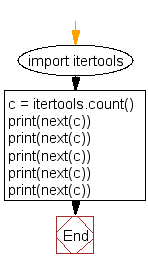﻿ Python: Create a list with infinite elements - w3resource

# Python: Create a list with infinite elements

## Python List: Exercise - 53 with Solution

Write a Python program to create a list with infinite elements.

Sample Solution:-

Python Code:

``````import itertools
c = itertools.count()
print(next(c))
print(next(c))
print(next(c))
print(next(c))
print(next(c))
```
```

Sample Output:

```0
1
2
3
4
```

Flowchart:## Visualize Python code execution:

The following tool visualize what the computer is doing step-by-step as it executes the said program:

Python Code Editor:

Have another way to solve this solution? Contribute your code (and comments) through Disqus.

What is the difficulty level of this exercise?

Test your Programming skills with w3resource's quiz.

﻿

## Python: Tips of the Day

Decapitalizes the first letter of a string:

Example:

```def tips_decapitalize(s, upper_rest=False):
return s[:1].lower() + (s[1:].upper() if upper_rest else s[1:])
print(tips_decapitalize('PythonTips'))
print(tips_decapitalize('PythonTips', True))
```

Output:

```pythonTips
pYTHONTIPS
```

We are closing our Disqus commenting system for some maintenanace issues. You may write to us at reach[at]yahoo[dot]com or visit us at Facebook